EE - 456 / E01 spring 2003

Advanced Communications Theory

Professor: Arida

Submitted By:

Andrew Buettner

Lab #3: Filtering

Wednesday, April 23, 2003

Table Of Contents

1) Cover Page 1

2) Table of Contents 2

3) Objective 4

4) Components Used 4

5) Procedures 4

6) Lab Data / Results 5

1) Diagram 1 5

2) Diagram 2 5

3) Diagram 3 6

4) Diagram 4 6

5) Diagram 5 7

6) Diagram 6 7

7) Diagram 7 8

8) Table 1 8

9) Table 2 8

10) Diagram 8 9

11) Diagram 9 9

12) Diagram 10 10

13) Diagram 11 10

14) Diagram 12 11

15) Diagram 13 11

16) Diagram 14 12

17) Diagram 15 12

18) Diagram 16 13

19) Diagram 17 13

20) Table 3 14

7 Answers to Lab Questions 14

7) Conclusions 14

Table Of Contents (Continued)

8) Attachments 14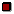Objective

The objective of this lab is to investigate the effects of filter simulation using digital signal processing. Both IIR and FIR filters will be employed.Components Used

1) Computer running Octave

2) Digital sampling board

3) Oscilloscope #01-00001

4) Function Generator #02-00006Procedures

1) Using Octave, enter "iir(1,1)"

2) Enter command "ssrat(100000)"

3) Set the function generator to generate a 1KHz sine wave with 2Vp
4) Observe the output, it should mimic the input.

5) Vary the frequency up to 50KHz, and observe the output; it should continue to mimic the input.

6) Create an array a = [1.0, 0.0, .1716]

7) Create an array b = [.2929, .5858, .2929]

8) Using these coefficients, analytically solve and plot the transfer function for this filter.

9) Compute the expected frequency response at w = 0, ws/8, ws/4, and 3ws/8

10) Plot the resulting expected frequency response.

11) Use the command "iirp(b, a)" to plot the resulting frequency response.

12) Implement the filter using "iir(b, a)"

13) Set the function generator to 1KHz.

14) Observe the frequency response as the frequency is increased form 1KHz to 50KHz.

15) Using several points, estimate the frequency response.

16) Create an array b = [.5, .5].

17) Compute and plot the frequency response to this filter.

18) Use "firp(b)" to plot the frequency response.

19) Implement the filter using "fir(b)"

20) Repeat steps 13 to 15 to estimate the frequency response.

21) Create an array a = [0, 0, 1, 1, 1, 1, 0, 0] to simulate a band pass filter.

22) Enter command "f=0:7;"

23) Enter command "f=f/7;"

24) Using the remez command, and the newly generated f and a arrays, create an array b to simulate a 60 pole "brick wall" filter.

25) Plot this FIR filter.

26) Implement the filter.

27) Repeat steps 13 through 15 to estimate the frequency response.Lab Data / Results

1) Diagram 1: 1KHz sine wave with no filtering:2) Diagram 2: 21KHz sine wave with no filtering:3) Diagram 3: Expected frequency response for IIR filter: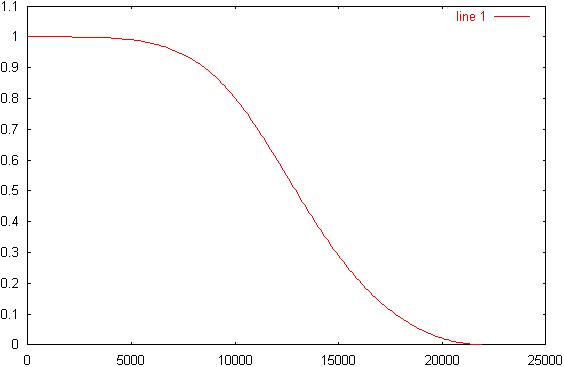4) Diagram 4: 1KHz filtered sine wave: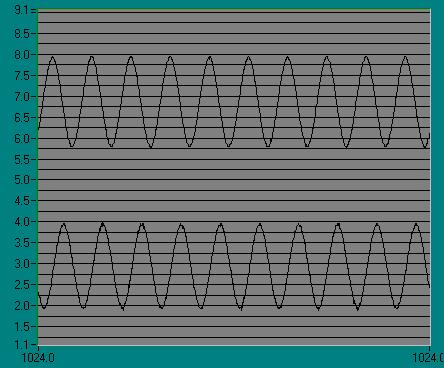5) Diagram 5: 5.5KHz filtered sine wave: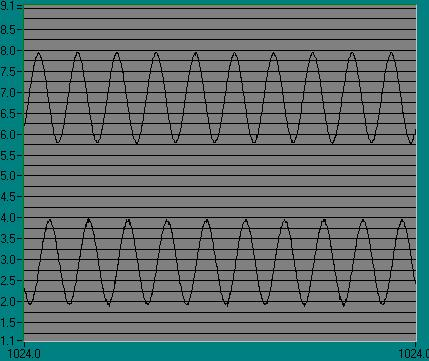6) Diagram 6: 11KHz filtered sine wave:7) Diagram 7: 16.5KHz filtered sine wave: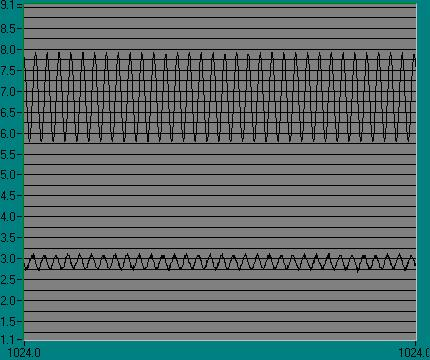8) Table 1: Expected vs. observed frequency response for IIR filter:

Frequency:

1KHz

5.5KHz

11KHz

16.5KHz

Expected:

1.0

.98

.76

.20

Observed:

.94

.91

.65

.17

%error:

6.0%

7.1%

14.5%

15.0%

9) Table 2: Expected vs. observed frequency response for FIR filter:

Frequency:

1KHz

5.5KHz

11KHz

16.5KHz

Expected:

1.0

.91

.73

.44

Observed:

.97

.88

.67

.37

%error:

3.0%

3.3%

8.2%

15.9%

10) Diagram 8: Expected frequency response for FIR filter: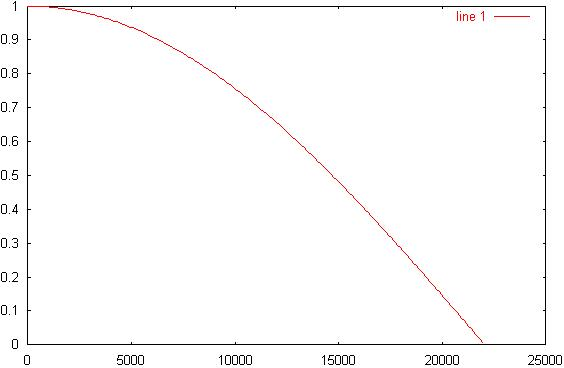11) Diagram 9: 1KHz filtered sine wave: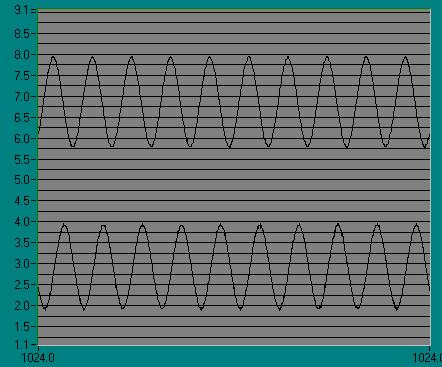12) Diagram 10: 5.5KHz filtered sine wave: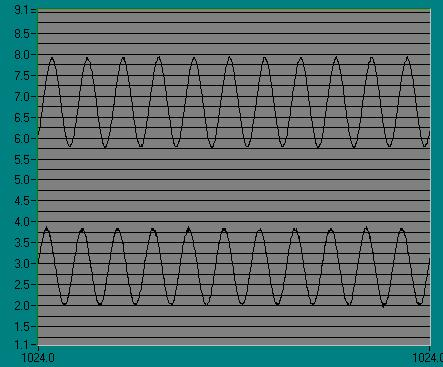13) Diagram 11: 11KHz filtered sine wave:14) Diagram 12: 16.5KHz filtered sine wave: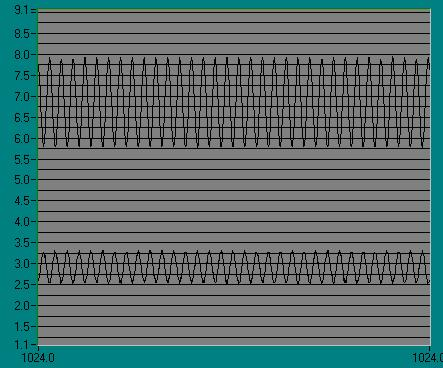15) Diagram 13: Octave frequency plot for rimez filter: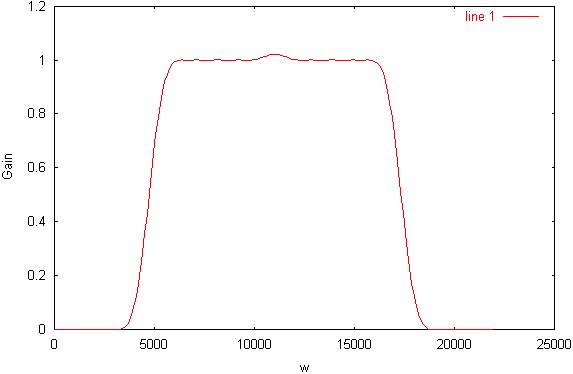16) Diagram 14: 1KHz filtered sine wave: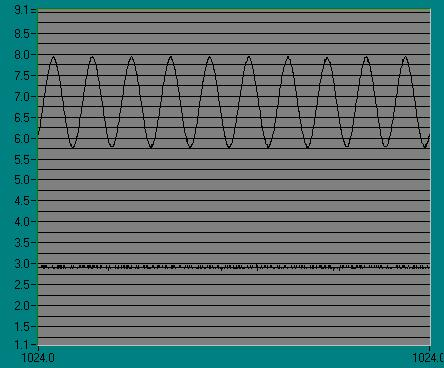17) Diagram 15: 5.5KHz filtered sine wave: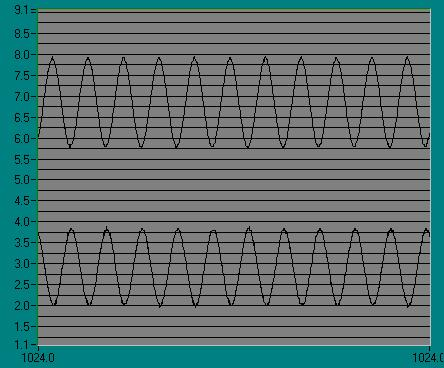18) Diagram 16: 11KHz filtered sine wave: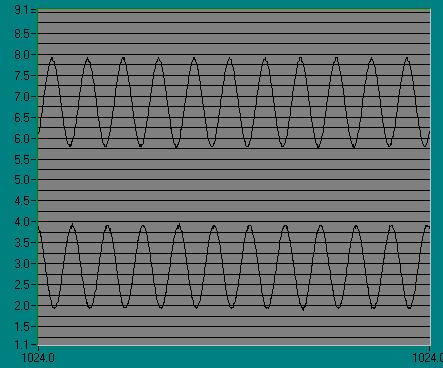19) Diagram 17: 16.5KHz filtered sine wave:20) Table 3: Estimated frequency response for remez filter:

Frequency:

1KHz

5.5KHz

11KHz

16.5KHz

Expected:

0

1

1.1

1

Observed:

0

.88

.95

.84

%error:

0%

12.0%

13.6%

16.0%Answers to Lab Questions

1) Q: What sequence of MatLab® commands would be required to implement a fourth order Butterworth low-pass filter whose cutoff frequency is ws/6?

A: 1) a = [e^((-ws/6) * T/2) * cos((2^(1/2) / 2) * (ws/6) * T) + (1/2) * sin((2^(1/2) / 2) * (ws / 6) * T), 0];

2) b = [2 * e^((-ws/6) * T / 2) * cos((2^(1/2) / 2 * (ws/6) * T), e^((ws/6) * T)];

3) iir(b,a)

2) Q: We wish to cascade two FIR filters with coefficients b1 and b2 using only one DSP. What sequence of MatLab® commands can accomplish this?

A: 1) b3 = conv(b1, b2);

2) fir(b3)

3) Q: What happens to the frequency response of an IIR filter as the poles approach the unit circle?

A: The cutoff frequency increases, and the system will begin to ring.Conclusions

This lab has demonstrated the effects of digital filtering on an signal. A majority of the error shown in Tables 1, 2, and 3 is the result of poor estimation of the expected gain and weight should not be as heavy on these values. Data in the lab was set up to compensate for a sampling rate of 44100. All data observed was based on this rate. Because a board with fixed sampling rate was used, the ssrat(100000) instruction was not used.Attachments

1. Original lab handout

2. Original lab data

3. Calculations

4. Octave® log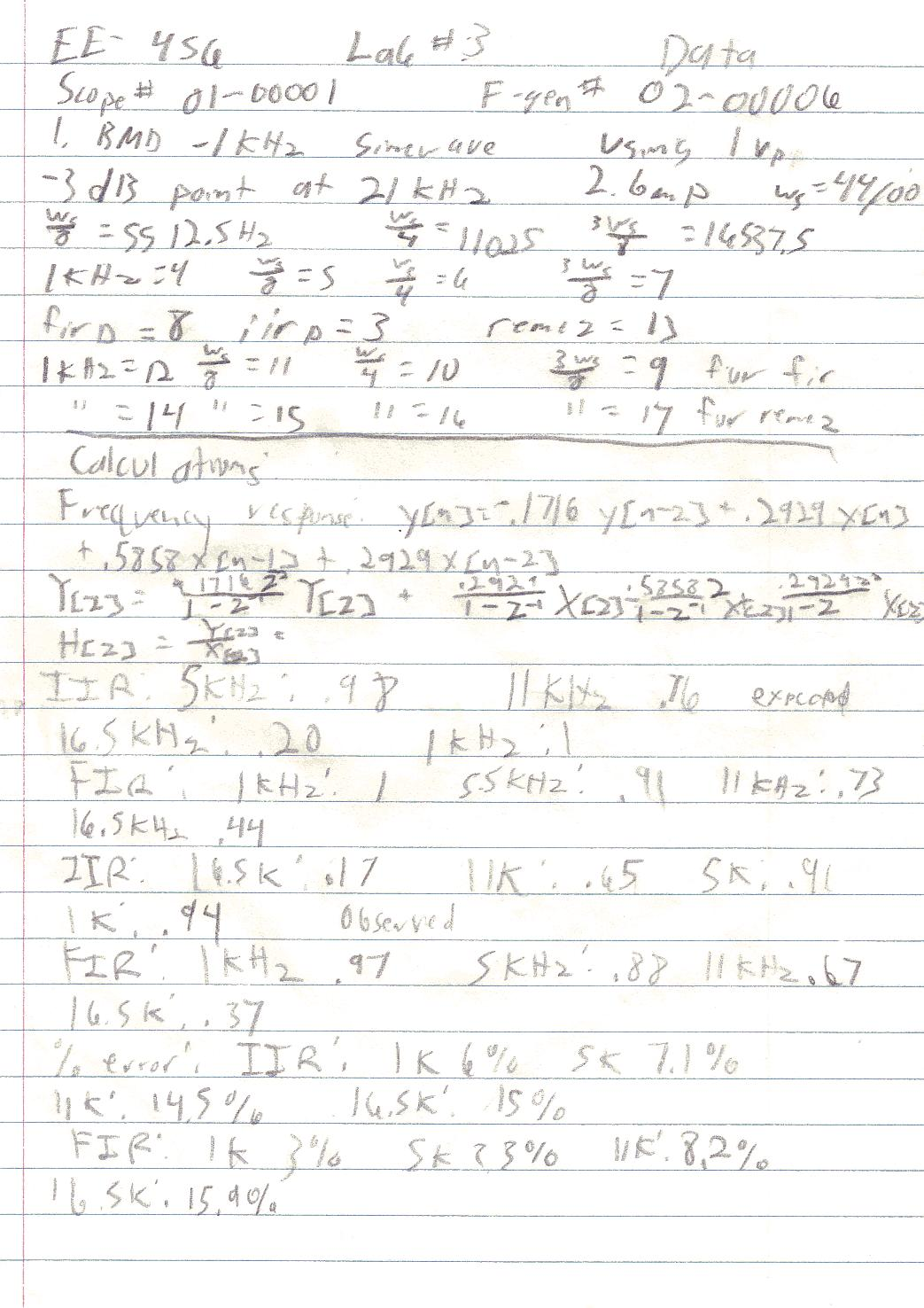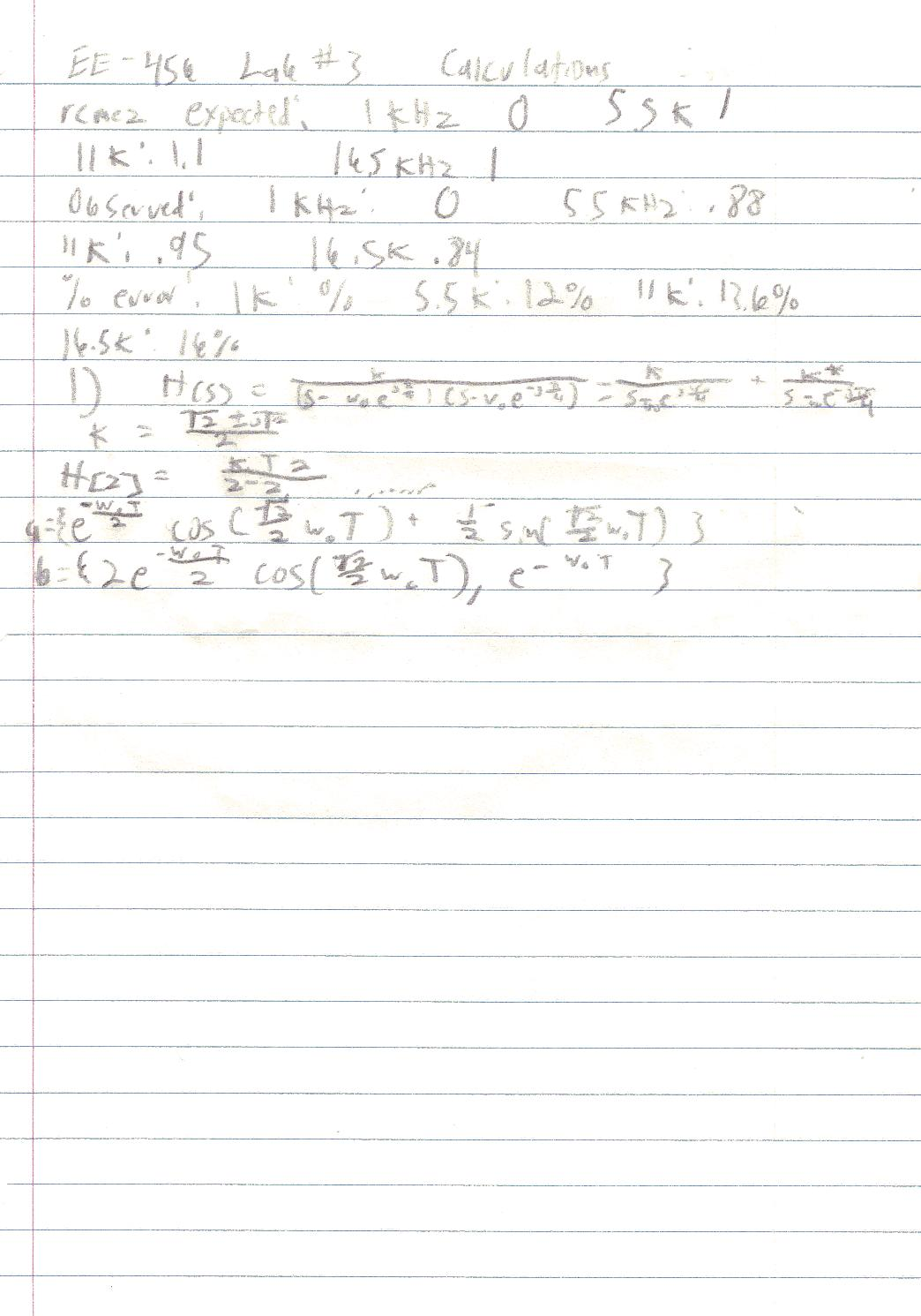GNU Octave, version 2.1.31 (i586-pc-cygwin).

Copyright (C) 1996, 1997, 1998, 1999, 2000 John W. Eaton.

This is free software with ABSOLUTELY NO WARRANTY.

For details, type `warranty'.

*** This is a development version of Octave. Development releases

*** are provided for people who want to help test, debug, and improve

*** Octave.

***

*** If you want a stable, well-tested version of Octave, you should be

*** using one of the stable releases (when this development release

*** was made, the latest stable version was 2.0.16).

octave:1> fir(1)

1 coefficients

Max = 1.000000

Scale factor = 4.000000

Scale shift = 2

float hex

b = 1.0000 4000

octave:2> iir(1,1)

m = 1

b = 1.000000

n = 1

a = 1.000000

1 coefficients

Max = 1.000000

Scale factor = 4.000000

Scale shift = 2

float hex

b = 1.0000 4000

a = 1.0000 cccccccc

octave:3>

octave:3> a=[1,0,.1716];

octave:4> b=[.2929,.5858,.2929]

b =

0.29290 0.58580 0.29290

octave:5> iirp(b,a)

octave:6> iir(b,a)

m = 3

b = 0.292900

b = 0.585800

b = 0.292900

n = 3

a = 1.000000

a = 0.000000

a = 0.171600

3 coefficients

Max = 0.585800

Scale factor = 2.000000

Scale shift = 1

float hex

b = 0.2929 257d

b = 0.5858 4afb

b = 0.2929 257d

a = 1.0000 cccccccc

a = 0.0000 0

a = 0.1716 ffffea0a

octave:7> b=[.5,.5];

octave:8> firp(b)

octave:9> fir(b)

2 coefficients

Max = 0.500000

Scale factor = 2.000000

Scale shift = 1

float hex

b = 0.5000 4000

b = 0.5000 4000

octave:10> a=[0,1,1,0];

octave:11> f=0:3;

octave:12> f=f/3;

octave:13> f=0:7;

octave:14> f=f/7;

octave:15> a=[0,0,1,1,1,1,0,0];

octave:16> b=remez(60,f,a);

octave:17> firp(b)

octave:18> fir(b)

61 coefficients

Max = 0.572051

Scale factor = 2.000000

Scale shift = 1

float hex

b = -0.0007 ffffffe9

b = -0.0000 0

b = 0.0001 4

b = -0.0000 0

b = 0.0018 3b

b = 0.0000 0

b = 0.0020 40

b = 0.0000 0

b = -0.0047 ffffff66

b = -0.0000 0

b = -0.0062 ffffff34

b = -0.0000 0

b = 0.0046 95

b = -0.0000 0

b = 0.0179 249

b = 0.0000 0

b = -0.0005 fffffff1

b = 0.0000 0

b = -0.0321 fffffbe5

b = -0.0000 0

b = -0.0216 fffffd3e

b = -0.0000 0

b = 0.0519 6a3

b = 0.0000 0

b = 0.0736 96b

b = 0.0000 0

b = -0.0643 fffff7c7

b = 0.0000 0

b = -0.3078 ffffd89a

b = -0.0000 0

b = 0.5721 4938

b = -0.0000 0

b = -0.3078 ffffd89a

b = 0.0000 0

b = -0.0643 fffff7c7

b = 0.0000 0

b = 0.0736 96b

b = 0.0000 0

b = 0.0519 6a3

b = -0.0000 0

b = -0.0216 fffffd3e

b = -0.0000 0

b = -0.0321 fffffbe5

b = 0.0000 0

b = -0.0005 fffffff1

b = 0.0000 0

b = 0.0179 249

b = -0.0000 0

b = 0.0046 95

b = -0.0000 0

b = -0.0062 ffffff34

b = -0.0000 0

b = -0.0047 ffffff66

b = 0.0000 0

b = 0.0020 40

b = 0.0000 0

b = 0.0018 3b

b = -0.0000 0

b = 0.0001 4

b = -0.0000 0

b = -0.0007 ffffffe9

octave:19>

octave:19> warning: connection to external plotter (pid = 1027) lost --

warning: please try your plot command(s) again

octave:19>

octave:19>